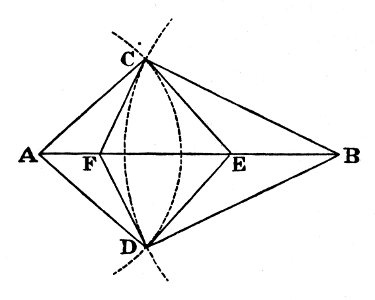#Pillow-Problems: Problem #13

Math Lair Home > Source Material > Pillow-Problems > Problem #13

## Problem:

13.

Given the lengths of the radii of two intersecting Circles, and the distance between their centres: find the area of the Tetragon formed by the tangents at the points of intersection.

[3/89

13.

If ‘2M’ = area of Tetragon whose vertices are the Centres and the Points of intersection; and if its sides be ‘a’, ‘b’, and its diagonal, joining the Centres, ‘c’: required area

=
 32M³ (b² + c² − a²) · (c² + a² − b²)
.

## Solution:

13.Let A, B, be the centres of the Circles; C, D their points of intersection; and CFDE the Tetragon whose area is required.

Let the sides of the Triangle ABC be a, b, c; and its ∠s α, β, γ.

Then CE = b · tan α, and CF = a · tan β.

Also ∠FCE = ∠ACE + ∠FCB - γ = π − γ;

∴ sin FCE = sin γ.

Hence area of Triangle FCE = ½ · ab · tan α · tan β · sin γ;

∴ area of Tetragon =
 ab sin α sin β sin γ cos α cos β
.

Now, writing ‘M’ for area of Triangle ABC, we have

sin α =
 2M bc
, sin β =
 2M ca
, sin γ =
 2M ab
;

∴ area of Tetragon = ab ·
 8M³ a²b²c²
·
 4bc · ca (b² + c² − a²) · (c² + a² − b²)

=
 32M³ (b² + c² − a²) · (c² + a² − b²)
.

Q.E.F.